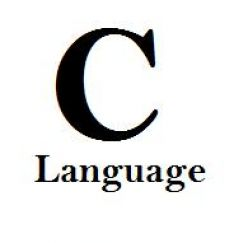# Quiz 5 - Functions And Pointers

10 Questions | Attempts: 117
ShareSettingsClass BEE-2A

• 1.
Pointer is a special kind of a variable which is used to store _______ of a variable
• A.

Data Type

• B.

Value

• C.

Variable name

• D.

• 2.
The operator used to declare a pointer variable is
• A.

^

• B.

%

• C.

*

• D.

&

• 3.
Variable passing by reference is achieved by using pointers.
• A.

True

• B.

False

• 4.
Char data type uses _____ byte (s), int data type uses _____ byte (s) and float uses _____ byte (s)
• A.

2, 4, 1

• B.

4, 2, 1

• C.

1, 2, 4

• D.

4, 1, 2

• 5.
Passing by value means an exact copy of the variable is created and the value is assigned to it.
• A.

True

• B.

False

• 6.
Choose the best answerPrior to using a pointer variable
• A.

It should be declared

• B.

It should be initialized

• C.

It should be both declared and initialized

• D.

None of the above

• 7.
What does the following mean?int *ptr, p;
• A.

Ptr is a integer pointer, p is not

• B.

Both ptr and p are integer pointers

• C.

P may be a pointer but ptr is not

• D.

Both ptr and p are not pointers

• 8.
A pointer is
• A.

A keyword used to create variables

• B.

A variable that stores address of an instruction

• C.

A variable that stores address of other variable

• D.

All of the above

• 9.
#include<stdio.h>void f(){    printf("hello");}void main(){   f();}the code above has an error.
• A.

True

• B.

False

• 10.
Suppose a variable x has  an address fff0. declaring a pointer and assigning address of x is as follow.int *p = &x;printf("address of x = %d", *p);the output will be [address of x = fff0] stored in pointer p?
• A.

True

• B.

False

## Related TopicsBack to top
×

Wait!
Here's an interesting quiz for you.